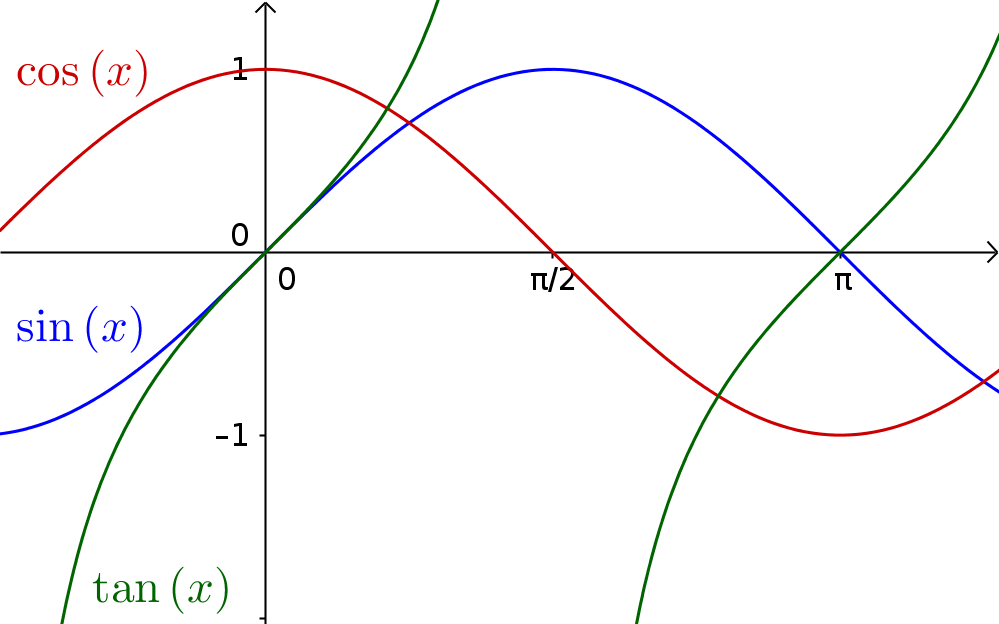Math Real functions Non-rational functions

# Non-rational functions

In non-rational functions , besides the rational ones, there are also additional arithmetic operations (for example square roots, logharithms, etc.).

You should know the following non-rational functions:

 Square root functionsTrigonometric functionsExponential functionsLogarithmic functions### Examples

Other examples of non-rational functions are:

• $f(x)=5\cdot\sin(x)$

• $f(x)=\sqrt{x}$

• $f(x)=12\cdot2^{x-1}$